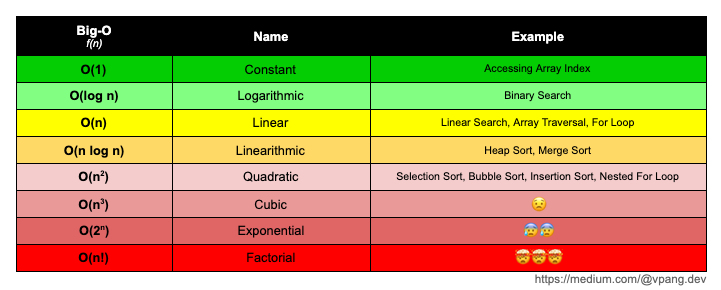# Asymptotic Runtime Complexity — Analyzing Time and SpaceMy definition of Big-O Notation.

In computational theory, the efficiency and performance of an algorithm can be mathematically quantified by comparing its’ arbitrary input size against the runtime. However, an identical algorithm (or even a program) being executed on one computer may not necessarily have the same runtime on another computer. Dependencies such as processor speed, I/O speed, cache size, and etc. are without a doubt contributing factors. As such, the fundamentals behind this theory of analysis is asymptotic in nature.

Asymptotic (asymptote) is defined by their ratio as a given variable approaches infinity.

Take the Golden Ratio (φ) for example. Given its algebraic formula,

`(a+b)/a = a/b = φ`

this ratio eventually converges “asymptotically” to 1.61803398874989484820 as it reaches infinity.

However, that’s beside the point.

The main idea here is that, an asymptotic analysis of an algorithm or program considers all factors as constants other than its’ arbitrary input size. And as a software developer, it is essential to understand and consider an algorithms’ runtime complexity not only for the purposes of efficiency, but ultimately the scalability.

# What is Asymptotic Notations?

Asymptotic Notations are basically mathematical expressions used for representing an algorithm’s rate of growth in terms of its input size. For example, this can be expressed by a function f(n) where n is the input.

These notations are commonly categorized as follows:

• Upper Bound (O / o)
• Lower Bound (Ω / ω)
• Average Bound (Θ)

Upper Bound
The (tight) upper bound is what is most commonly known as the Big-O Notation; while the loose upper bound is expressed with Little-o. Both of these essentially represent an algorithm’s worst case , and is a measure of the longest possible runtime.

Lower Bound
The lower bound is expressed with Big-Omega (Ω) and Little-Omega (ω); where as ω is the measure of the loose lower bound. As you may have guessed, this represents an algorithm’s best case, and is a measure of the shortest possible runtime.

Average Bound
The average bound is a measure of an algorithm’s average runtime, and is expressed with Theta (Θ).

Note: Tight bound is a more accurate representation of runtime complexity, whereas loose bound is not.

# Why Big-O Notation?

Although every asymptotic notation (O, o, Ω , ω, Θ) are useful in representing runtime, Big-O Notation is more widely used. This is because we as developers are more concerned about an algorithm’s worst case, as we typically want to make sure that a given algorithm will never exceed a specific upper bound. Hence, the benchmarking of the Big-O Notation.

# A Simple Guide to Big-O Notation

Utilizing the diagram below as a visual guide for runtime complexities in Big-O Notation, everything above `O(n log n)` are not very scalable, and should be avoided.If your algorithm is O(n²) or greater, it’s time to reconsider and start refactoring.

# Calculating the Big-O

Examples:

`// O(1)function(str){   console.log(str)};// O(n)function printArray(arr){   for(let i = 0; i < arr.length; i++){      ...   }};// O(n log n)function sumOfTwoNum (arr, sum){   const sortedArr = arr.sort(( a , b ) => a - b);   let left = 0;   let right = arr.length - 1;   while (left !== right){       ...   }};// O(n^2)function iterateArray(arr){   for(let i = 0; i < arr.length; i++){      for(let j = 0; j < arr.length; j++){         ...      }   }};// O(2^n)function fib(num){   if(num < 2){      return num   }   return fib(num - 1) + fib(num - 2)};`

*Ignore the Constants*
In asymptotic analysis we are assessing the runtime based on arbitrary data size of `n`. Therefore, since constants do not change regardless of data size, we can simply ignore them.

`const reverseString = (str) => {    let splitStr = str.split('')                     //O(n)    let reverseStr = splitStr.reverse().join('')     //O(n)    return reverseStr}`

In the example above, the Big-O is `O(2n)`. Since we ignore constants, we can ignore `2`, leaving us with just `O(n)`.

*Ignore the lesser significant terms*
Keep in mind we are working with Big-O notation (not theta/omega), and our primary concern here in our calculation is the “worst case” scenario.

Take the below function for example:

`const iterateArray = (arr) => {   for(let i = 0; i < arr.length; i++){      //O(n)      ...   }      for(let i = 0; i < arr.length; i++){      //O(n^2) = O(n) * O(n)      for(let j = 0; j < arr.length; j++){         ...      }   }};`

We know the first `for` loop is `O(n)`, while the the nested `for` loop is `O(n^2)`. Since Big-O is only concerned about worst case, `O(n)` is the less significant term compared to `O(n^2)`; therefore our Big-O in this case would be `O(n^2)`.

The study surrounding asymptotic analysis is without a doubt, far more vaster than what this article could cover. Therefore, other implementation(s) of asymptotic analysis, such as when dealing with `n` dimensional arrays, vectors, and other data structures, are not duly covered in this article.

With that being said, I hope you find this article helpful in understanding the fundamentals behind the concept of asymptotic analysis; and more specifically why Big-O Notation is often leveraged.

If you’ve found this helpful, please let me know.

## More from Vincent Pang

https://www.linkedin.com/in/vincentpangu